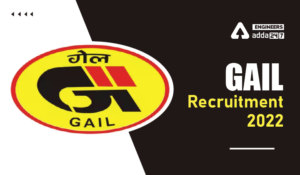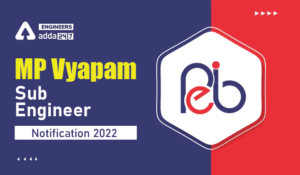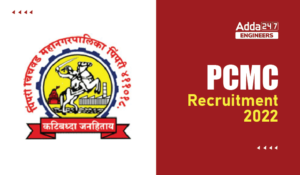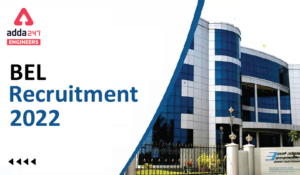Engineering Jobs   »   Quiz: Mechanical Engineering 28 April 2020

# Quiz: Mechanical Engineering 28 April 2020

Quiz: Mechanical Engineering
Exam: ISRO (TECHNICAL ASST.)
Topic: Miscellaneous

Each question carries 4 mark.
Negative marking: 1 mark
Time: 15 Minute

Q1. Lower critical temperature (in deg C) of steel is
(a) 950
(b) 1560
(c) 800
(d) 723

Q2. Reynolds’s number is the ratio of
(a) Thermal conductivity to kinematic viscosity
(b) Inertia force to viscous force
(c) Heat convected to heat conducted
(d) None of these

Q3. Efficiency of Carnot cycle is (Where,T1 and T2 are the highest temperature and lowest temperature during the cycle)
(a) (T1/T2) -1
(b) 1-(T2/T1)
(c) (T1/T2) +1
(d) T1/T2

Q4. When the Mach number is less than unity, then the flow is
(a) Supersonic
(b) Sonic
(c) Subsonic
(d) None of the above

Q5. The moment of inertia of a circular section of diameter D about its diameter is________the moment of inertia about the polar axis.
(a) four times
(b) double
(c) three times
(d) half

Q6. Which of the following material cannot be machined by EDM?
(a) Steel
(b) Cast iron
(c) Titanium
(d) Glass

Q7. The locus of a point on a string unwound from a circular disc is
(a) A circle
(b) A cycloid
(c) A parabola
(d) An involute

Q8. The heat that must be absorbed by ice of mass 500g at -10 deg C to take it to water at 20 deg C is (specific heat of ice is 2.2 kJ/kg-K, specific heat of the water is 4.2 kJ/kg-K and latent heat of fusion of ice is 300kJ/kg)
(a) 53 kJ
(b) 243 kJ
(c) 192 kJ
(d) 203 kJ

Q9. There are five numbers 2, 3, 4, 5 and 6. The average value and root mean square value of these numbers are
(a) 4, 4
(b) 4, 4.242
(c) 5, 4
(d) 4, 5

Q10. Maximum power transmission in a belt is met when the total tension in the belt equals
(a) centrifugal tension
(b) 2π times centrifugal tension
(c) thrice the centrifugal tension
(d) half the centrifugal tension

Solutions

S1. Ans(d)
Sol. Lower critical temperature of steel is 723 deg C and higher critical temperature of steel is 925 deg C.

S2. Ans(b)
Sol. Reynolds’s Number(Re) = (Inertia Force)/(Viscous Force)=(ρVd)/μ
Here,
ρ=Density of fluid
V=Average velocity of fluid
d=Diameter of conduit
μ=Dynamic viscosity of fluid

S3. Ans(b)
Sol. Efficiency of Carnot cycle (η)=1-(T2/T1)

S4. Ans(c)
Sol.
1. If Mach Number (Ma<1) then flow is subsonic.
2. If Mach Number (Ma=1) then flow is sonic.
3. If Mach Number (Ma>1) then flow is supersonic.
4. If Mach Number (Ma>3) then flow is hyper-sonic.

S5. Ans(d)
Sol.
Moment of inertia about the diameter=(πD^4)/64
Moment of inertia about polar axis=(πD^4)/32

S6. Ans(d)
Sol. Glass is non-conductive material, so it cannot be machined by EDM process.

S7. Ans(d)
Sol. The locus of a point on a string unwound from a circular disc is an involute.

S8. Ans(d)
Sol. Heat absorbed by ice at -10 deg C to convert into water 20 deg C = heat taken by ice from-10 deg C to 0 deg C + heat taken for phase change of ice into water at 0 deg C + heat taken by water from 0 deg C to 20 deg C
=0.5×2.2×(0-(-10)+0.5×300+0.5×4.2×(20-0)
=203 kJ

S9. Ans(b)
Sol.
Average value=(Sum of elements)/(Total number of elements)=(2+3+4+5+6)/5=4
Root mean square value=√((2^2+3^2+4^2+5^2+6^2)/5)=4.242

S10. Ans(c)
Sol.
For maximum power transmission in a belt,
Total tension in the belt (T)=3×Centrifugal Tension (Tc)

Sharing is caring!

Thank You, Your details have been submitted we will get back to you.
•HPSC Lecturer Result 2022,
•How to Prepare for SSC JE 2022 General A...
•GAIL Recruitment 2022, GAIL 282 Non Exec...
•MP Vyapam Sub Engineer Notification 2022...
•PCMC Recruitment 2022, Check here the de...
•BEL Recruitment 2022, Check here For 13 ...